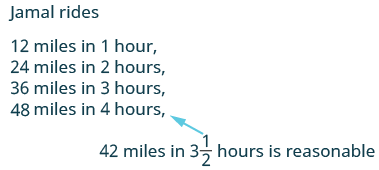Using the Distance, Rate, and Time Formula

Learning Outcomes

• Use the problem-solving method to solve problems using the distance, rate, and time formula

One formula you’ll use often in algebra and in everyday life is the formula for distance traveled by an object moving at a constant speed. The basic idea is probably already familiar to you. Do you know what distance you traveled if you drove at a steady rate of $60$ miles per hour for $2$ hours? (This might happen if you use your car’s cruise control while driving on the Interstate.) If you said $120$ miles, you already know how to use this formula!

The math to calculate the distance might look like this:

$\begin{array}{}\\ \text{distance}=\left(\Large\frac{60\text{ miles}}{1\text{ hour}}\normalsize\right)\left(2\text{ hours}\right)\hfill \\ \text{distance}=120\text{ miles}\hfill \end{array}$

In general, the formula relating distance, rate, and time is

$\text{distance}\text{=}\text{rate}\cdot \text{time}$

Distance, Rate, and Time

For an object moving at a uniform (constant) rate, the distance traveled, the elapsed time, and the rate are related by the formula

$d=rt$

where $d=$ distance, $r=$ rate, and $t=$ time.

Notice that the units we used above for the rate were miles per hour, which we can write as a ratio $\Large\frac{miles}{hour}$. Then when we multiplied by the time, in hours, the common units “hour” divided out. The answer was in miles.

example

Jamal rides his bike at a uniform rate of $12$ miles per hour for $3\Large\frac{1}{2}$ hours. How much distance has he traveled?

Solution:

 Step 1. Read the problem.You may want to create a mini-chart to summarize the information in the problem. $d=?$$r=12\text{mph}$ $t=3 \Large\frac{1}{2}\normalsize\text{hours}$ Step 2. Identify what you are looking for. distance traveled Step 3. Name. Choose a variable to represent it. let d = distance Step 4. Translate.Write the appropriate formula for the situation. Substitute in the given information. $d=rt$$d=12\cdot 3\Large\frac{1}{2}$ Step 5. Solve the equation. $d=42\text{ miles}$ Step 6. Check: Does 42 miles make sense?Step 7. Answer the question with a complete sentence. Jamal rode 42 miles.

try it

In the following video we provide another example of how to solve for distance given rate and time.

Example

Rey is planning to drive from his house in San Diego to visit his grandmother in Sacramento, a distance of $520$ miles. If he can drive at a steady rate of $65$ miles per hour, how many hours will the trip take?

try it

In the following video we show another example of how to find rate given distance and time.